NCERT Solutions Class 10 Maths Chapter 1 Real Numbers are provided here. These solutions are prepared by our expert faculties to help students in their first term exam preparations. They solve and provide the NCERT Solutions for Maths so as to aid the students to solve the problems easily. They also focus on preparing the solutions in such a way that it is easy to understand for the students. They provide a detailed and step-wise explanation of each answer to the questions given in the exercises of NCERT books.

Answers for the questions present in Real Numbers are given in the first chapter of Maths Solutions of NCERT Class 10. Here, students are introduced to a lot of important concepts that will be useful for those who wish to pursue mathematics as a subject in their Class 11. Based on these solutions of NCERT, students can prepare for their upcoming first term. These solutions are helpful as it is in accordance with the term-wise CBSE Syllabus for 2021-22.

## Exercise 1.1 Page: 7

1. Use Euclid’s division algorithm to find the HCF of:

i. 135 and 225

ii. 196 and 38220

iii. 867 and 255

Solutions:

i. 135 and 225

As you can see, from the question 225 is greater than 135. Therefore, by Euclid’s division algorithm, we have,

225 = 135 × 1 + 90

Now, remainder 90 ≠ 0, thus again using division lemma for 90, we get,

135 = 90 × 1 + 45

Again, 45 ≠ 0, repeating the above step for 45, we get,

90 = 45 × 2 + 0

The remainder is now zero, so our method stops here. Since, in the last step, the divisor is 45, therefore, HCF (225,135) = HCF (135, 90) = HCF (90, 45) = 45.

Hence, the HCF of 225 and 135 is 45.

ii. 196 and 38220

In this given question, 38220>196, therefore the by applying Euclid’s division algorithm and taking 38220 as divisor, we get,

38220 = 196 × 195 + 0

We have already got the remainder as 0 here. Therefore, HCF(196, 38220) = 196.

Hence, the HCF of 196 and 38220 is 196.

iii. 867 and 255

As we know, 867 is greater than 255. Let us apply now Euclid’s division algorithm on 867, to get,

867 = 255 × 3 + 102

Remainder 102 ≠ 0, therefore taking 255 as divisor and applying the division lemma method, we get,

255 = 102 × 2 + 51

Again, 51 ≠ 0. Now 102 is the new divisor, so repeating the same step we get,

102 = 51 × 2 + 0

The remainder is now zero, so our procedure stops here. Since, in the last step, the divisor is 51, therefore, HCF (867,255) = HCF(255,102) = HCF(102,51) = 51.

Hence, the HCF of 867 and 255 is 51.

2. Show that any positive odd integer is of the form 6q + 1, or 6q + 3, or 6q + 5, where q is some integer.

Solution:

Let a be any positive integer and b = 6. Then, by Euclid’s algorithm, a = 6q + r, for some integer q ≥ 0, and r = 0, 1, 2, 3, 4, 5, because 0≤r<6.

Now substituting the value of r, we get,

If r = 0, then a = 6q

Similarly, for r= 1, 2, 3, 4 and 5, the value of a is 6q+1, 6q+2, 6q+3, 6q+4 and 6q+5, respectively.

If a = 6q, 6q+2, 6q+4, then a is an even number and divisible by 2. A positive integer can be either even or odd Therefore, any positive odd integer is of the form of 6q+1, 6q+3 and 6q+5, where q is some integer.

3. An army contingent of 616 members is to march behind an army band of 32 members in a parade. The two groups are to march in the same number of columns. What is the maximum number of columns in which they can march?

Solution:

Given,

Number of army contingent members=616

Number of army band members = 32

If the two groups have to march in the same column, we have to find out the highest common factor between the two groups. HCF(616, 32), gives the maximum number of columns in which they can march.

By Using Euclid’s algorithm to find their HCF, we get,

Since, 616>32, therefore,

616 = 32 × 19 + 8

Since, 8 ≠ 0, therefore, taking 32 as new divisor, we have,

32 = 8 × 4 + 0

Now we have got remainder as 0, therefore, HCF (616, 32) = 8.

Hence, the maximum number of columns in which they can march is 8.

4. Use Euclid’s division lemma to show that the square of any positive integer is either of the form 3m or 3m + 1 for some integer m.

Solutions:

Let x be any positive integer and y = 3.

By Euclid’s division algorithm, then,

x = 3q + r for some integer q≥0 and r = 0, 1, 2, as r ≥ 0 and r < 3.

Therefore, x = 3q, 3q+1 and 3q+2

Now as per the question given, by squaring both the sides, we get,

x2 = (3q)2 = 9q2 = 3 × 3q2

Let 3q2 = m

Therefore, x2= 3m ……………………..(1)

x= (3q + 1)= (3q)2+12+2×3q×1 = 9q2 + 1 +6q = 3(3q2+2q) +1

Substitute, 3q2+2q = m, to get,

x2= 3m + 1 ……………………………. (2)

x2= (3q + 2)= (3q)2+22+2×3q×2 = 9q+ 4 + 12q = 3 (3q+ 4q + 1)+1

Again, substitute, 3q2+4q+1 = m, to get,

x2= 3m + 1…………………………… (3)

Hence, from equation 1, 2 and 3, we can say that, the square of any positive integer is either of the form 3m or 3m + 1 for some integer m.

5. Use Euclid’s division lemma to show that the cube of any positive integer is of the form 9m, 9m + 1 or 9m + 8.

Solution:

Let x be any positive integer and y = 3.

By Euclid’s division algorithm, then,

x = 3q+r, where q≥0 and r = 0, 1, 2, as r ≥ 0 and r < 3.

Therefore, putting the value of r, we get,

x = 3q

or

x = 3q + 1

or

x = 3q + 2

Now, by taking the cube of all the three above expressions, we get,

Case (i): When r = 0, then,

x2= (3q)3 = 27q3= 9(3q3)= 9m; where m = 3q3

Case (ii): When r = 1, then,

x3 = (3q+1)3 = (3q)+13+3×3q×1(3q+1) = 27q3+1+27q2+9q

Taking 9 as common factor, we get,

x= 9(3q3+3q2+q)+1

Putting = m, we get,

Putting (3q3+3q2+q) = m, we get ,

x3 = 9m+1

Case (iii): When r = 2, then,

x3 = (3q+2)3= (3q)3+23+3×3q×2(3q+2) = 27q3+54q2+36q+8

Taking 9 as common factor, we get,

x3=9(3q3+6q2+4q)+8

Putting (3q3+6q2+4q) = m, we get ,

x3 = 9m+8

Therefore, from all the three cases explained above, it is proved that the cube of any positive integer is of the form 9m, 9m + 1 or 9m + 8.

## Exercise 1.2 Page: 11

1. Express each number as a product of its prime factors:

(i) 140

(ii) 156

(iii) 3825

(iv) 5005

(v) 7429

Solutions:

(i) 140

By Taking the LCM of 140, we will get the product of its prime factor.

Therefore, 140 = 2 × 2 × 5 × 7 × 1 = 22×5×7

(ii) 156

By Taking the LCM of 156, we will get the product of its prime factor.

Hence, 156 = 2 × 2 × 13 × 3 × 1 = 22× 13 × 3

(iii) 3825

By Taking the LCM of 3825, we will get the product of its prime factor.

Hence, 3825 = 3 × 3 × 5 × 5 × 17 × 1 = 32×52×17

(iv) 5005

By Taking the LCM of 5005, we will get the product of its prime factor.

Hence, 5005 = 5 × 7 × 11 × 13 × 1 = 5 × 7 × 11 × 13

(v) 7429

By Taking the LCM of 7429, we will get the product of its prime factor.

Hence, 7429 = 17 × 19 × 23 × 1 = 17 × 19 × 23

2. Find the LCM and HCF of the following pairs of integers and verify that LCM × HCF = product of the two numbers.

(i) 26 and 91

(ii) 510 and 92

(iii) 336 and 54

Solutions:

(i) 26 and 91

Expressing 26 and 91 as product of its prime factors, we get,

26 = 2 × 13 × 1

91 = 7 × 13 × 1

Therefore, LCM (26, 91) = 2 × 7 × 13 × 1 = 182

And HCF (26, 91) = 13

Verification

Now, product of 26 and 91 = 26 × 91 = 2366

And Product of LCM and HCF = 182 × 13 = 2366

Hence, LCM × HCF = product of the 26 and 91.

(ii) 510 and 92

Expressing 510 and 92 as product of its prime factors, we get,

510 = 2 × 3 × 17 × 5 × 1

92 = 2 × 2 × 23 × 1

Therefore, LCM(510, 92) = 2 × 2 × 3 × 5 × 17 × 23 = 23460

And HCF (510, 92) = 2

Verification

Now, product of 510 and 92 = 510 × 92 = 46920

And Product of LCM and HCF = 23460 × 2 = 46920

Hence, LCM × HCF = product of the 510 and 92.

(iii) 336 and 54

Expressing 336 and 54 as product of its prime factors, we get,

336 = 2 × 2 × 2 × 2 × 7 × 3 × 1

54 = 2 × 3 × 3 × 3 × 1

Therefore, LCM(336, 54) = = 3024

And HCF(336, 54) = 2×3 = 6

Verification

Now, product of 336 and 54 = 336 × 54 = 18,144

And Product of LCM and HCF = 3024 × 6 = 18,144

Hence, LCM × HCF = product of the 336 and 54.

3. Find the LCM and HCF of the following integers by applying the prime factorisation method.

(i) 12, 15 and 21

(ii) 17, 23 and 29

(iii) 8, 9 and 25

Solutions:

(i) 12, 15 and 21

Writing the product of prime factors for all the three numbers, we get,

12=2×2×3

15=5×3

21=7×3

Therefore,

HCF(12,15,21) = 3

LCM(12,15,21) = 2 × 2 × 3 × 5 × 7 = 420

(ii) 17, 23 and 29

Writing the product of prime factors for all the three numbers, we get,

17=17×1

23=23×1

29=29×1

Therefore,

HCF(17,23,29) = 1

LCM(17,23,29) = 17 × 23 × 29 = 11339

(iii) 8, 9 and 25

Writing the product of prime factors for all the three numbers, we get,

8=2×2×2×1

9=3×3×1

25=5×5×1

Therefore,

HCF(8,9,25)=1

LCM(8,9,25) = 2×2×2×3×3×5×5 = 1800

4. Given that HCF (306, 657) = 9, find LCM (306, 657).

Solution: As we know that,

HCF×LCM=Product of the two given numbers

Therefore,

9 × LCM = 306 × 657

LCM = (306×657)/9 = 22338

Hence, LCM(306,657) = 22338

5. Check whether 6n can end with the digit 0 for any natural number n.

Solution: If the number 6n ends with the digit zero (0), then it should be divisible by 5, as we know any number with unit place as 0 or 5 is divisible by 5.

Prime factorization of 6n = (2×3)n

Therefore, the prime factorization of 6n doesn’t contain prime number 5.

Hence, it is clear that for any natural number n, 6is not divisible by 5 and thus it proves that 6n cannot end with the digit 0 for any natural number n.

6. Explain why 7 × 11 × 13 + 13 and 7 × 6 × 5 × 4 × 3 × 2 × 1 + 5 are composite numbers.

Solution: By the definition of composite number, we know, if a number is composite, then it means it has factors other than 1 and itself. Therefore, for the given expression;

7 × 11 × 13 + 13

Taking 13 as common factor, we get,

=13(7×11×1+1) = 13(77+1) = 13×78 = 13×3×2×13

Hence, 7 × 11 × 13 + 13 is a composite number.

Now let’s take the other number,

7 × 6 × 5 × 4 × 3 × 2 × 1 + 5

Taking 5 as a common factor, we get,

=5(7×6×4×3×2×1+1) = 5(1008+1) = 5×1009

Hence, 7 × 6 × 5 × 4 × 3 × 2 × 1 + 5 is a composite number.

7. There is a circular path around a sports field. Sonia takes 18 minutes to drive one round of the field, while Ravi takes 12 minutes for the same. Suppose they both start at the same point and at the same time, and go in the same direction. After how many minutes will they meet again at the starting point?

Solution: Since, Both Sonia and Ravi move in the same direction and at the same time, the method to find the time when they will be meeting again at the starting point is LCM of 18 and 12.

Therefore, LCM(18,12) = 2×3×3×2×1=36

Hence, Sonia and Ravi will meet again at the starting point after 36 minutes.

## Exercise 1.3 Page: 14

1. Prove that √is irrational.

Solutions: Let us assume, that 5 is rational number.

i.e. 5 = x/y (where, x and y are co-primes)

y5= x

Squaring both the sides, we get,

(y5)2 = x2

⇒5y2 = x2……………………………….. (1)

Thus, x2 is divisible by 5, so x is also divisible by 5.

Let us say, x = 5k, for some value of k and substituting the value of x in equation (1), we get,

5y2 = (5k)2

⇒y2 = 5k2

is divisible by 5 it means y is divisible by 5.

Clearly, x and y are not co-primes. Thus, our assumption about 5 is rational is incorrect.

Hence, 5 is an irrational number.

2. Prove that 3 + 2√5 + is irrational.

Solutions: Let us assume 3 + 25 is rational.

Then we can find co-prime x and y (y ≠ 0) such that 3 + 2√5 = x/y

Rearranging, we get,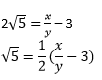Since, x and y are integers, thus,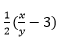is a rational number.

Therefore, 5 is also a rational number. But this contradicts the fact that 5 is irrational.

So, we conclude that 3 + 25 is irrational.

3. Prove that the following are irrationals:

(i) 1/√2

(ii) 7√5

(iii) 6 + 2

Solutions:

(i) 1/2

Let us assume 1/√2 is rational.

Then we can find co-prime x and y (y ≠ 0) such that 1/√2 = x/y

Rearranging, we get,

√2 = y/x

Since, x and y are integers, thus, √2 is a rational number, which contradicts the fact that √2 is irrational.

Hence, we can conclude that 1/√2 is irrational.

(ii) 75

Let us assume 7√5 is a rational number.

Then we can find co-prime a and b (b ≠ 0) such that 7√5 = x/y

Rearranging, we get,

√5 = x/7y

Since, x and y are integers, thus, √5 is a rational number, which contradicts the fact that √5 is irrational.

Hence, we can conclude that 7√5 is irrational.

(iii) 6 +2

Let us assume 6 +√2 is a rational number.

Then we can find co-primes x and y (y ≠ 0) such that 6 +√2 = x/y⋅

Rearranging, we get,

√2 = (x/y) – 6

Since, x and y are integers, thus (x/y) – 6 is a rational number and therefore, √2 is rational. This contradicts the fact that √2 is an irrational number.

Hence, we can conclude that 6 +√2 is irrational.

## Exercise 1.4 Page: 17

1. Without actually performing the long division, state whether the following rational numbers will have a terminating decimal expansion or a non-terminating repeating decimal expansion:

(i) 13/3125 (ii) 17/8 (iii) 64/455 (iv) 15/1600 (v) 29/343 (vi) 23/(2352) (vii) 129/(225775) (viii) 6/15 (ix) 35/50 (x) 77/210

Solutions:

Note: If the denominator has only factors of 2 and 5 or in the form of 2m ×5n then it has terminating decimal expansion.

If the denominator has factors other than 2 and 5 then it has a non-terminating decimal expansion.

(i) 13/3125

Factorizing the denominator, we get,

3125 = 5 × 5 × 5 × 5 × 5 = 55

Since, the denominator has only 5 as its factor, 13/3125 has a terminating decimal expansion.

(ii) 17/8

Factorizing the denominator, we get,

8 = 2×2×2 = 23

Since, the denominator has only 2 as its factor, 17/8 has a terminating decimal expansion.

(iii) 64/455

Factorizing the denominator, we get,

455 = 5×7×13

Since, the denominator is not in the form of 2m × 5n, thus 64/455 has a non-terminating decimal expansion.

(iv) 15/ 1600

Factorizing the denominator, we get,

1600 = 26×52

Since, the denominator is in the form of 2m × 5n, thus 15/1600 has a terminating decimal expansion.

(v) 29/343

Factorizing the denominator, we get,

343 = 7×7×7 = 73 Since, the denominator is not in the form of 2m × 5n thus 29/343 has a non-terminating decimal expansion.

(vi)23/(2352)

Clearly, the denominator is in the form of 2m × 5n.

Hence, 23/ (2352) has a terminating decimal expansion.

(vii) 129/(225775)

As you can see, the denominator is not in the form of 2m × 5n.

Hence, 129/ (225775) has a non-terminating decimal expansion.

(viii) 6/15

6/15 = 2/5

Since, the denominator has only 5 as its factor, thus, 6/15 has a terminating decimal expansion.

(ix) 35/50

35/50 = 7/10

Factorising the denominator, we get,

10 = 2 × 5

Since, the denominator is in the form of 2m × 5n thus, 35/50 has a terminating decimal expansion.

(x) 77/210

77/210 = (7× 11)/ (30 × 7) = 11/30

Factorising the denominator, we get,

30 = 2 × 3 × 5

As you can see, the denominator is not in the form of 2m × 5n .Hence, 77/210 has a non-terminating decimal expansion.

2. Write down the decimal expansions of those rational numbers in Question 1 above which have terminating decimal expansions.

Solutions:

(i) 13/312513/3125 = 0.00416

(ii) 17/817/8 = 2.125

(iii) 64/455 has a Non terminating decimal expansion

(iv)15/ 160015/1600 = 0.009375

(v) 29/ 343 has a Non terminating decimal expansion

(vi)23/ (2352) = 23/(8×25)= 23/20023/ (2352) = 0.115

(vii) 129/ (225775) has a Non terminating decimal expansion

(viii) 6/15 = 2/5(ix) 35/50 = 7/10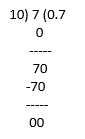35/50 = 0.7

(x) 77/210 has a non-terminating decimal expansion.

3. The following real numbers have decimal expansions as given below. In each case, decide whether they are rational or not. If they are rational, and of the form, p q what can you say about the prime factors of q?

(i) 43.123456789

(ii) 0.120120012000120000. . .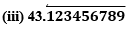Solutions:

(i) 43.123456789

Since it has a terminating decimal expansion, it is a rational number in the form of p/q and q has factors of 2 and 5 only.

(ii) 0.120120012000120000. . .

Since, it has non-terminating and non- repeating decimal expansion, it is an irrational number.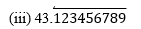Since it has non-terminating but repeating decimal expansion, it is a rational number in the form of p/q and q has factors other than 2 and 5.

## NCERT Solutions for Class 10 Maths Chapter 1- Real Numbers

Real Number is one of the important topics in Maths and it has a weightage of 6 marks in Class 10 (Unit – Number Systems) Maths first term exams. The average number of questions asked from this chapter is usually 3. Three questions were asked from this chapter in the previous year board examination (2018).

1. One out of three questions in part A (1 marks).
2. One out of three questions in part B (2 marks).
3. One out of three questions in part C (3 marks).

• Euclid’s Division Algorithm
• The Fundamental Theorem of Arithmetic
• Revisiting Rational & Irrational Numbers
• Decimal Expansions

List of Exercises in class 10 Maths Chapter 1:

Exercise 1.1 Solutions 5 Question ( 4 long, 1 short)
Exercise 1.2 Solutions 7 Question ( 4 long, 3 short)
Exercise 1.3 Solutions 3 Question ( 3 short)
Exercise 1.4 Solutions 3 Question ( 3 short)

Real Numbers is introduced in Class 9 and this is discussed more in detail in Class 10. NCERT Solutions for Class 10 Maths Chapter 1 Real Numbers provides the answers to the questions present in this chapter. The chapter discusses real numbers and their applications. The divisibility of integers using Euclid’s division algorithm says that any positive integer a can be divided by another positive integer b such that the remainder will be smaller than b. On the other hand, The Fundamental Theorem of Arithmetic works on multiplication of positive integers.

The chapter starts with the introduction of real numbers in section 1.1 followed by two very important topics in sections 1.2 and 1.3

• Euclid’s Division Algorithm – It includes 5 questions based on Theorem 1.1 – Euclid’s Division Lemma.
• The Fundamental Theorem of Arithmetic – Explore the applications of this topic which talks about the multiplication of positive integers, through solutions of the 7 problems in Exercise 1.2.

Next, it discusses the following topics which were introduced in Class 9.

• Revisiting Rational & Irrational Numbers – In this, the solutions for 3 problems in Exercise 1.3 are given which also use the topic in the last Exercise 1.2.
• Decimal Expansions – It explores when the decimal expansion of a rational number is terminating and when it is recurring. It includes a total of 3 problems with sub-parts in Exercise 1.4

### Key Features of NCERT Solutions for Class 10 Maths Chapter 1- Real Numbers

• These NCERT Solutions let you solve and revise the update CBSE syllabus of Class 10 for 2021-22.
• After going through the stepwise solutions given by our subject expert teachers, you will be able to score more marks.
• It follows NCERT guidelines which help in preparing the students accordingly.
• It contains all the important questions from the examination point of view.
• It helps in scoring well in maths in exams.

## Frequently Asked Questions on NCERT Solutions for Class 10 Maths Chapter 1

### What are the main topics covered in the NCERT Solutions for Class 10 Maths Chapter 1?

The main topics covered in the NCERT Solutions for Class 10 Maths Real Numbers are Euclid’s division algorithm, the fundamental theorem of arithmetic, revisiting rational & irrational numbers and decimal expansions. Based on these concepts, our experts created the solutions effectively. By giving importance to these concepts students can score good marks in class tests as well as in the Class 10 first term exam.

### How many exercises are there in NCERT Solutions for Class 10 Maths Chapter 1?

There are four exercises in the NCERT Solutions for Class 10 Maths Real Numbers. These Solutions of NCERT Maths Class 10 Chapter 1 help the students in solving the problems adroitly and efficiently. They also focus on cracking the Solutions of Maths in such a way that it is easy for the students to understand.

### Are NCERT Solutions for Class 10 Maths Chapter 1 important from the exam point of view?

Yes, the first chapter Real Numbers of NCERT Solutions for Class 10 is important from an exam perspective. It contains short answer questions as well as long answer questions to increase the ability of problem-solving. These solutions are created by SSC CGL APEX Coaching expert faculty to help students in the preparation of their examinations.